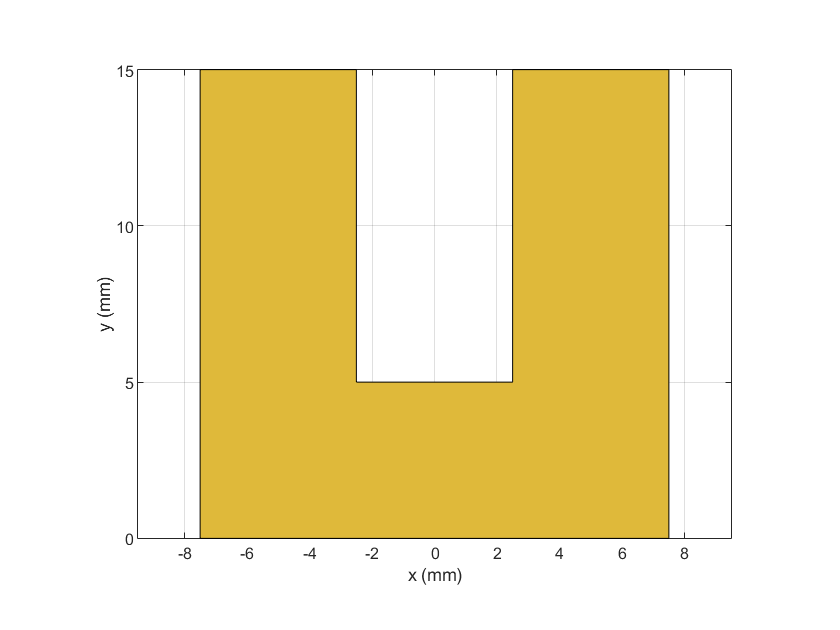ubendRightAngle

Create right-angle U-bend shape on X-Y plane

Description

Use the ubendRightAngle object to create a right-angle U-bend shape on the X-Y plane.

Creation

Description

example

bend = ubendRightAngle creates a right-angle U-bend shape on the X-Y plane.

bend = ubendRightAngle(Name=Value) sets Properties using one or more name-value arguments. For example, ubendRightAngle(ReferencePoint=[1 1]) creates a right-angle U-bend at the reference point [1 1]. Properties not specified retain their default values.

Properties

expand all

Name of the right-angle U-bend, specified as a character vector or a string scalar.

Example: bend = ubendRightAngle(Name="ubendrightangle1")

Data Types: char | string

Reference point of the right-angle U-bend in Cartesian coordinates, specified as a two-element vector.

Example: bend = ubendRightAngle(ReferencePoint=[1 2])

Data Types: double

Length of the right-angled U-bend in meters, specified as a three-element vector of positive elements.

Example: bend = ubendRightAngle(Length=[0.0250 0.0150 0.0250])

Data Types: double

Width of the right-angle U-bend in meters, specified as a three-element vector of positive values.

Example: bend = ubendRightAngle(Width=[0.0150 0.0150 0.0010])

Data Types: double

Object Functions

 add Boolean unite operation on two RF PCB shapes and Shape1 & Shape2 for RF PCB shapes area Calculate area of RF PCB shape in square meters intersect Boolean intersection operation on two RF PCB shapes mesh Change and view mesh properties of metal or dielectric in PCB component minus Shape1 - Shape2 for RF PCB shapes plus Shape1 + Shape2 for RF PCB shapes rotate Rotate RF PCB shape about defined axis rotateX Rotate RF PCB shape about x-axis rotateY Rotate RF PCB shape about y-axis and angle rotateZ Rotate RF PCB shape about z-axis subtract Boolean subtraction operation on two RF PCB shapes scale Change size of RF PCB shape by fixed amount show Display PCB component structure or PCB shape translate Move RF PCB shape to new location

Examples

collapse all

Create a right-angle U-bend with default properties.

bend = ubendRightAngle
bend =
ubendRightAngle with properties:

Name: 'myRightAngleubend'
ReferencePoint: [0 0]
Length: [0.0150 0.0050 0.0150]
Width: [0.0050 0.0050 0.0050]

View the shape.

show(bend)